Dr. J's Maths.com
Where the techniques of Maths
are explained in simple terms.

Trigonometry - Basic relationships.
Summary.

Use this summary to help you learn the results - all of which are important.

Cover up the third column with a page or your calculator, answer the question and then check.

NO CHEATING!!!

 Basic ratios.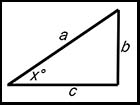sin x° =cos x° =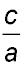tan x° =Reciprocal ratios. Remember.Think third letter. cosec x°=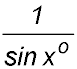sec x° =cot x° =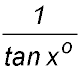Complementary results. sin (90° - x°) = cos x° cos (90° - x°) = sin x° tan (90 - x°) = cot x° Special result. tan x° =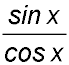Pythagorean results. sin2 x + cos2 x = 1 tan2 x + 1 = sec2 x 1 + cot2 x = cosec2 x